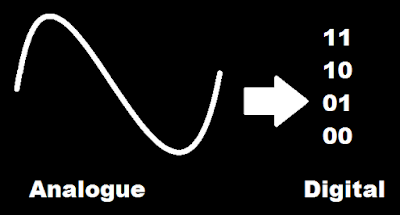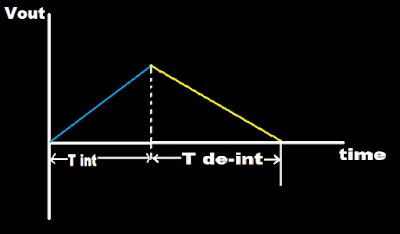# Integrating or Dual Slope ADC

55 views

+1 vote
by•There are basically 4 types of Analog to Digital converter (ADC).

4.Delta sigma converter

•Dual slope ADC is use for converting analog to digital value.

•This is integrator.

•Dual slope ADC is slow but have high accuracy and have high resistance to noise.

•This method is use in digital multimeter or digital voltmeter.

•It is basically have integrator, comparator, control logic, clock, counter and electronically controlled switches.

•Its operation requires two voltage slopes hence it is name as Dual slope.

•The unknown voltage is applied to Vin and Known but with negative polarity voltage is applied to Vref.

•Vin is for fixed amount of time and Vref is applied for variable amount of time.

•One slope (/) is made due to unknown voltage.

•The negative polarity voltage is then applied until it become zero means second slope (\) will form.

•The comparator is use to detect zero crossing.

•Capacitor charges and discharges linearly with a constant slopes.

•T de-int is more than T int because discharging time of capacitor is more than its charging time.

•If T de-int is large means large voltage and if T de-int is less means less voltage.

•When the switch is at Vin position capacitor charges (charging time of capacitor is less).

•And when the switch is at Vref position capacitor discharges (charging time of capacitor is more).

1. Single slope/Voltage to frequency or integrating type A/D converter

2. Dual slope integrating type A/D converter

Single slope/Voltage to frequency or integrating type A/D converter:

Explanation: An analog voltage can be converted to digital form, by producing pulses whose frequency is proportional to the analog voltage. These pulses are counted by a counter for a fixed duration and the reading of the counter will be proportional to the frequency of the pulses and hence, to the analog voltage. Voltage-to-frequency converter In these types of A/D converters, the voltage is converted (by a voltage-to-frequency converter) into a set of pulses repetition rate (or frequency) is proportional to the magnitude of the input. The pulses are counted by an electronic counter similar to the way the number of wavelengths was counted by the time-interval counter in the ramp-type DVM.

or

Dual slope Integration A/D converter

Explanation: In this ADC, an unknown analog voltage and a known reference voltage are converted into equivalent time periods using an integrator, These time periods are measured by the counter. This circuit is called dual slope ADC because the analog voltage and reference voltage are converted to ramp signals of different slopes by the integrator. The dc voltage to be converted by the dual-slope converter, Vin, is fed to an integrator, which produces a ramp waveform output, The ramp signal starts at zero and creases for a fixed time interval, T1 equal to the maximum count of the multiplied by the clock frequency. An 8-bit counter operating at 1 MHz would there by cause T1 to be 8 µs. The slope of the ramp is proportional to the magnitude of Vin. The end of the interval, T1 the carry-out (CO) bit of the ripple counter causes the switch to move to the - VREF position. In this position, a constant current (- VREFI R) begins to discharge capacitor C. The ripple counter is reset to zero there is a Co. The count continues until the zero crossing detector switches state as result of capacitor C being discharged. The counter is stopped by the zero crossing detector, and the resultant count is proportional to the input voltage. In the following derivation it is important to observe that t, is independent of the value of Rand C.

## Related questions

Explain the basic dual slope ADC technique used in digital multimeters with its diagram and measurement cycle.

Which type of ADC gives high resolution?

Describe the various blocks and their functions in pipelined ADC architecture.

Why energy meter is integrating type measuring instrument?

The number of comparators required in a 4 bit comparator type ADC is (A) 16 (B) 15 (C) 17 (D) 12

What is Analog to Digital Converter (ADC) ?

Which is the fastest type of analogue to digital converter?

Two sinusoidal signals of the same frequency are displayed on a dual-trace oscilloscope. One complete cycle of each signal covers 6 cm of the horizontal scale and the starting point of the horizontal scale and the starting point of the two signals are separated by 0.5 cm. The phase difference between the two signals in degrees is  (a) 30 (b) 45 (c) 60 (d) 90

In a dual trace oscilloscope, the display appears segmented when  (a) low frequency signals are observed in Alternate mode (b) low frequency signals are observed in Chop mode (c) high frequency signals are observed in Alternate mode (d) high frequency signals are observed in Chop mode

Number of comparators required for an 8 bit flash ADC is: (1) 8 (2) 16 (3) 255 (4) 256

A microprocessor is a _______ chip integrating all the functions of a CPU of a computer.  A. multiple B. single C. double D. triple

Integrating principle in the digital measurement is the conversion of   (a) Voltage to time (b) Voltage to frequency (c) Voltage to current (d) Current to voltage

An integrating digital voltmeter measures  A) true average value B) rms value C) peak value D) peak to peak value

When the initial slope of input sine wave is greater than the slew rate of an OPAMP, the output: (1) Has no offset (2) Approaches to triangular waveshape (3) Is pure sinusoidal (4) Is square wave

The set of transistor characteristics which enable α to be determined directly from the slope is (a) CB transfer characteristics (b) CE transfer characteristics (c) CB input characteristics (d) CE output characteristics

The four-quadrant dual operation requires  (a) Two full converters in series  (b) Two full converters connected back to back  (c) Two full converters connected in parallel.  (d) Two semiconverters connected back to back

A system has 14 poles and 2 zeros in its open -loop transfer function. The slope of its highest frequency asymptote in its magnitude plot is (a) - 40 dB/dec (b) - 240 dB/dec (c) + 40 dB/dec (d) + 240 dB/dec

A motor load system is stable when the slope of the torque speed characteristics of the motor is (a) higher than the slope of the torque speed characteristics of the load (b) lower than the slope of the torque speed characteristics of the load (c) zero (d) none of the above

In a dual converter, the circulating current   (a) allows smooth reversal of load current but increases the response time. (b) allows smooth reversal of load current with improved speed of response. (c) does not allow smooth reversal of load current but reduces response time. (d) flows only if there is no interconnecting conductor.

For a type three-control system the asymptote at lower frequency will have a slope  A) – 6 db per octave B) – 18 db per octave C) – 20 db per octave D) – 60 db per octave

Which among the following is the dual of Boolean expression X+YZ=(X+Y) (X+Z)?  (A) X(Y+Z)=XY+YZ (B) X.(Y+Z)=X.Y+X.Z (C) X+(Y+Z) = X.Y+Z (D) X+(YZ) = X+Y+Z

List the types of ADCs. Explain working principle of any one type of ADC.

If the range of an analog transducer is 0 to 10 V, then for a resolution of 5 mV, the bits of ADC will be _____.

Which of the following ADC has highest accuracy ?  (a) Successive approximation type (b) Flash or parallel type (c) Single slope integration type (d) Dual slope integration type

The slowest ADC among the following is : (A) Flash type (B) Successive approximation type (C) Integrating type (D) Counting type

An eight bit digital data 10101100 is fed to an ADC. The reference voltage is +10V. The analog output voltage will be:  (1) 1.05V (2) 6.74V (3) 10.10V (4) 5.15V

A 12-bit ADC is operating with a lus clock period and total conversion time is seen to be 14us always. The ADC must be of the type  a. Flash type b. Counting type c. Integrating type d. Successive approximation type

A flash type ADC uses  A) Counter B) Op—amps C) An integrator D) Flip flop

For an ADC, full scale output voltage is about 10 V.For a resolution of about 9.78mV, number of bits required is  A) 6 B) 8 C) 10 D) 12

In 8 bit successive approximation ADC, clock frequency is 1MHz and reference voltage is 10 V.Conversion time in µS for 5 V input is  A) 4 B) 8 C) 10 D) None of these

What is essential for a successive approximation Nbit ADC?  (A) 2N clock pulses for conversion, an up-down counter and a DAC. (B) N clock pulses for conversion, a binary counter and a comparator. (C) 2N clock pulses for conversion and a binary counter only. (D) N clock pulses for conversion, an up-down counter and a DAC.

In a sample-and-hold circuit, the aperture time is (A) time required following a sample (B) time required for the switch to open (C) transition time interval between sample and hold (D) time from the hold command to the opening of the switch

How many non-overlapping channels are available in IEEE 802.11b

In D/A converter, the resolution required is 50mv and the total maximum input is 10v. The number of bits required is   (A) 7 (B) 8 (C) 9 (D) 200

Choose the correct statement  (a) Digital multimeters are built using current measuring elements, while analog multimeters are built using voltage measuring units (b) Digital multimeters are built using voltage measuring units, while analog multimeters are built using current measuring units (c) Both digital and analog multimeters are built using voltage measuring units (d) Both digital and analog multimeters are built using current measuring units

An analog voltmeter has a sensitivity of 10kΩ/volt. The galvanometer used in constructing the instrument will produce a full scale deflection when the current passed through it is  (a) 10 mA (b) 20 mA (c) 50 mA (d) 100 μA

An optical fiber cable laid underground has developed a discontinuity at a distance d from the source end. The fault can be located using the instrument  (a) Optical spectrum Analyzer (OSA) (b) Optical Time Domain Reflectometer (OTDR) (c) Optical Power Meter (OPM) (d) Laser Diffractometer (LD)

Transient signals can be observed using  (a) storage oscilloscope (b) sampling oscilloscope (c) wave analyzer (d) spectrum analyzer

The trace on an oscilloscope continually moves to the right of the screen when  (a) the sweep is triggered. (b) the sweep period is larger than the signal period. (c) the sweep period is smaller than the signal period. (d) there is no weep.

To distinguish between signals having very close values, we need an instrument with  (a) high accuracy (b) high resolution (c) high sensitivity (d) high linearity

In a weighted-resistor digital-to-analog converter with 8-bit input, if the resistance corresponding to the MSB is 2K, what will be the resistance corresponding to the LSB?  (a) 250 Ω (d) 512 KΩ (c) 256 KΩ (d) 25.5 Ω

ESD stands for